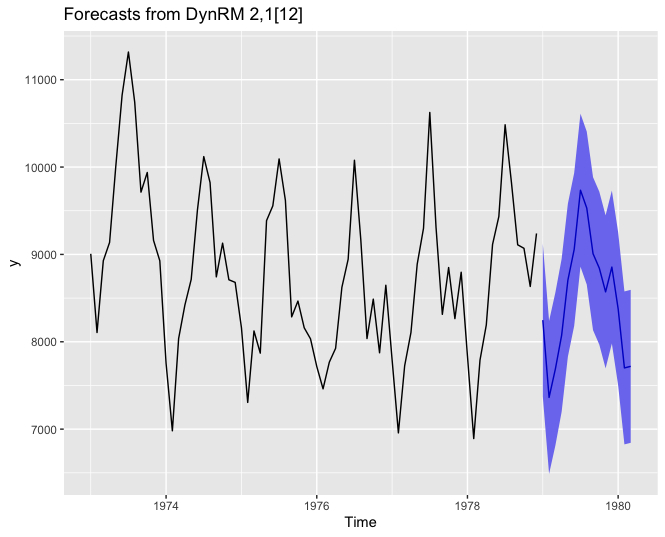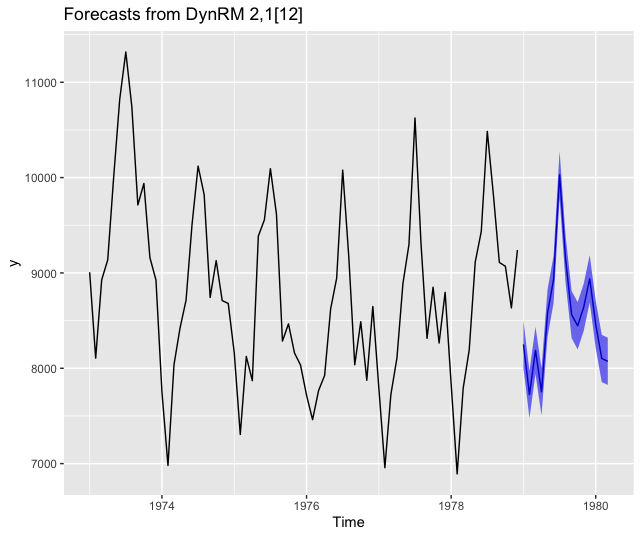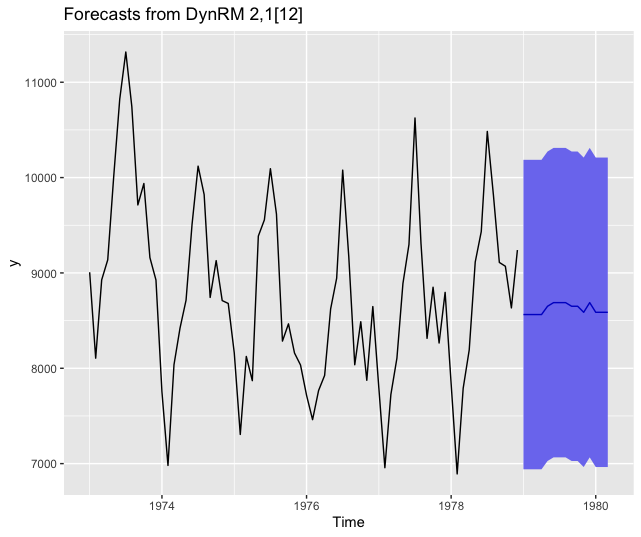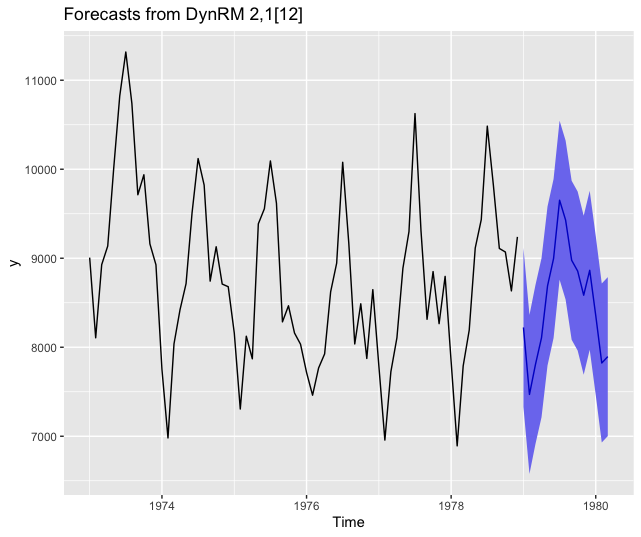Today, we examine some nontrivial use cases for ahead::dynrmfforecasting. Indeed, the examples presented in the package’s README work quite smoothly – for randomForest::randomForest and e1071::svm – because:

• the fitting function can handle matricial inputs (can be called as fitting_func(x, y), also said to have a x/y interface), and not only a formula input (can be called as fitting_func(y ~ ., data=df), the formula interface)

• the predict functions associated to randomForest::randomForest and e1071::svm do have a prototype like predict(object, newx) or predict(object, newdata), which are both well-understood input formats for ahead::dynrmf.

After reading this post, you’ll know how to adjust hundreds of different Statistical/Machine Learning (ML) models to univariate time series, and you’ll get a better understanding of how ahead::dynrmf works. If you’re not familiar with package ahead yet, you should read the following posts first:

The demo uses ahead::dynrmf in conjunction with R packages:

• ranger: random forests

• xgboost: gradient boosted decision trees

• caret: functions to streamline the model training process for complex regression problems.

# Installing package ahead

options(repos = c(
techtonique = 'https://techtonique.r-universe.dev',
CRAN = 'https://cloud.r-project.org'))



# Packages required for the demo

library(ahead)
library(forecast)
library(ranger)
library(xgboost)
library(caret)
library(gbm)
library(ggplot2)


# Forecasting using ahead::dynrmf’s default parameters

# ridge ------------------------------------------------------------------

# default, with ridge regression's regularization parameter minimizing GCV
autoplot(z)# Forecasting using ahead::dynrmf and ranger

# ranger ------------------------------------------------------------------

fit_func <- function(x, y, ...)
{
df <- data.frame(y=y, x) # naming of columns is mandatory for predict
ranger::ranger(y ~ ., data=df, ...)
}

predict_func <- function(obj, newx)
{
colnames(newx) <- paste0("X", 1:ncol(newx)) # mandatory, linked to df in fit_func
predict(object=obj, data=newx)\$predictions # only accepts a named newx
}

z <- ahead::dynrmf(USAccDeaths, h=15, level=95, fit_func = fit_func,
fit_params = list(num.trees = 500),
predict_func = predict_func)
autoplot(z)# Forecasting using ahead::dynrmf and xgboost

# xgboost -----------------------------------------------------------------

fit_func <- function(x, y, ...) xgboost::xgboost(data = x, label = y, ...)

z <- ahead::dynrmf(USAccDeaths, h=15, level=95, fit_func = fit_func,
fit_params = list(nrounds = 10,
verbose = FALSE),
predict_func = predict)
autoplot(z)# Forecasting using ahead::dynrmf and gbm through caret’s unified interface

# caret gbm -----------------------------------------------------------------

# unified interface, with hundreds of regression models
# https://topepo.github.io/caret/available-models.html

fit_func <- function(x, y, ...)
{
df <- data.frame(y=y, x)

caret::train(y ~ ., data=df,
method = "gbm",
trControl=caret::trainControl(method = "none"), # no cv
verbose = FALSE,
tuneGrid=data.frame(...))
}

predict_func <- function(obj, newx)
{
colnames(newx) <- paste0("X", 1:ncol(newx))
caret::predict.train(object=obj, newdata=newx, type = "raw")
}

z <- ahead::dynrmf(USAccDeaths, h=15, level=95, fit_func = fit_func,
fit_params = list(n.trees=10, shrinkage=0.01,
interaction.depth = 1,
n.minobsinnode = 10),
predict_func = predict_func)
autoplot(z)# Forecasting using ahead::dynrmf and glmnet through caret’s unified interface

# caret glmnet -----------------------------------------------------------------

# unified interface, with hundreds of regression models
# https://topepo.github.io/caret/available-models.html

fit_func <- function(x, y, ...)
{
df <- data.frame(y=y, x)

caret::train(y ~ ., data=df,
method = "glmnet",
trControl=caret::trainControl(method = "none"), # no cv
verbose = FALSE,
tuneGrid=data.frame(...))
}

predict_func <- function(obj, newx)
{
colnames(newx) <- paste0("X", 1:ncol(newx))
caret::predict.train(object=obj, newdata=newx, type = "raw")
}

z <- ahead::dynrmf(USAccDeaths, h=15, level=95, fit_func = fit_func,
fit_params = list(alpha=0.5, lambda=0.1),
predict_func = predict_func)
autoplot(z)